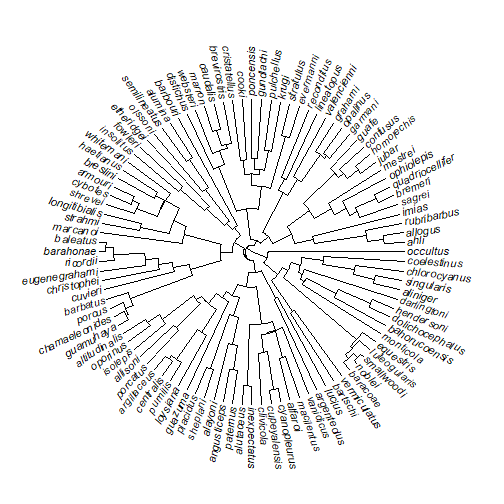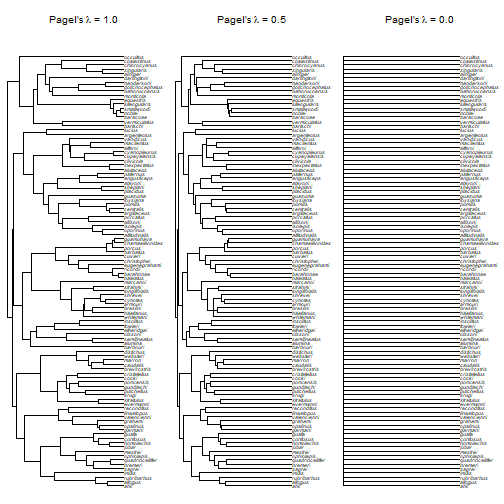Exercise 5: Models of continuous character evolution

In this tutorial, we will learn how to fit & compare alternative models for continuous character evolution for a single continuous character trait.

We will start by estimating 'phylogenetic signal,' a very simple & commonly-used measure of the tendency of related species to resemble one-another. Then, we will learn how to fit & compare a series of alternative evolutionary models for continuous traits.

Phylogenetic signal

First, start by loading the packages & data that we will use for the present exercise:

``````## packages
library(phytools)
``````
``````## Loading required package: ape
``````
``````## Loading required package: maps
``````
``````library(geiger)
``````

We will use data for body size & a phylogeny of 100 Anolis lizard species from the Caribbean. The files are the same ones we have used already, but they can be downloaded here:

First check to make sure the two files are in your current working directory, and then read them from file:

``````anole.tree<-read.tree("Anolis.tre")
``````

Let's examine the objects we have created. First, the tree:

``````anole.tree
``````
``````##
## Phylogenetic tree with 100 tips and 99 internal nodes.
##
## Tip labels:
##  ahli, allogus, rubribarbus, imias, sagrei, bremeri, ...
##
## Rooted; includes branch lengths.
``````
``````plotTree(anole.tree,fsize=0.9,ftype="i",type="fan",lwd=1)
```````obj` is a data frame. We will extract the column `"SVL"` and convert it to a simple vector.

``````## convert to a vector:
svl<-setNames(obj\$SVL,rownames(obj))
svl
``````
``````##            ahli         alayoni         alfaroi        aliniger
##         4.03913         3.81570         3.52665         4.03656
##        allisoni         allogus   altitudinalis         alumina
##         4.37539         4.04014         3.84299         3.58894
##       alutaceus     angusticeps     argenteolus     argillaceus
##         3.55489         3.78860         3.97131         3.75787
##         armouri   bahorucoensis        baleatus        baracoae
##         4.12168         3.82745         5.05306         5.04278
##       barahonae        barbatus        barbouri        bartschi
##         5.07696         5.00395         3.66393         4.28055
##         bremeri        breslini    brevirostris        caudalis
##         4.11337         4.05111         3.87415         3.91174
##       centralis  chamaeleonides    chlorocyanus     christophei
##         3.69794         5.04235         4.27545         3.88465
##       clivicola     coelestinus        confusus           cooki
##         3.75873         4.29797         3.93844         4.09154
##    cristatellus    cupeyalensis         cuvieri    cyanopleurus
##         4.18982         3.46201         4.87501         3.63016
##         cybotes     darlingtoni       distichus dolichocephalus
##         4.21098         4.30204         3.92880         3.90855
##       equestris      etheridgei   eugenegrahami       evermanni
##         5.11399         3.65799         4.12850         4.16561
##         fowleri         garmani         grahami           guafe
##         4.28878         4.76947         4.15427         3.87746
##       guamuhaya         guazuma       gundlachi       haetianus
##         5.03695         3.76388         4.18810         4.31654
##      hendersoni      homolechis           imias    inexpectatus
##         3.85983         4.03281         4.09969         3.53744
##       insolitus        isolepis           jubar           krugi
##         3.80047         3.65709         3.95260         3.88650
##      lineatopus   longitibialis        loysiana          lucius
##         4.12861         4.24210         3.70124         4.19891
##    luteogularis      macilentus        marcanoi          marron
##         5.10109         3.71576         4.07948         3.83181
##         mestrei       monticola          noblei        occultus
##         3.98715         3.77061         5.08347         3.66305
##         olssoni        opalinus      ophiolepis        oporinus
##         3.79390         3.83838         3.63796         3.84567
##        paternus        placidus       poncensis        porcatus
##         3.80296         3.77397         3.82038         4.25899
##          porcus      pulchellus         pumilis quadriocellifer
##         5.03803         3.79902         3.46686         3.90162
##      reconditus        ricordii     rubribarbus          sagrei
##         4.48261         5.01396         4.07847         4.06716
##    semilineatus        sheplani         shrevei      singularis
##         3.69663         3.68292         3.98300         4.05800
##      smallwoodi         strahmi       stratulus     valencienni
##         5.03510         4.27427         3.86988         4.32152
##       vanidicus    vermiculatus        websteri       whitemani
##         3.62621         4.80285         3.91655         4.09748
``````

Let's conduct two main tests for phylogenetic signal using the anole body size data. The first test is Blomberg’s K, which compares the variance of PICs to what we would expect under a Brownian motion model. K = 1 means that relatives resemble one another as much as we should expect under BM; K < 1 means that there is less “phylogenetic signal” than expected under BM, while K > 1 means that there is more. A significant p-value returned from `phylosig` tells you that there is significant phylogenetic signal - that is, close relatives are more similar than random pairs of species.

``````phylosig(anole.tree,svl,test=TRUE)
``````
``````## \$K
##  1.553679
##
## \$P
##  0.001
``````

Another method for testing phylogenetic signal is Pagel’s λ. λ is a scaling coefficient for the expected covariances between species. One way to interpret this is as a tree transformation that stretches tip branches (and thus the predicted variances between species) relative to internal branches (and predicted covariances). λ close to zero, means phylogenetic signal equivalent to that expected if the data arose on a star phylogeny (that is, no phylogenetic signal). λ = 1 corresponds to a Brownian motion model; 0 < λ < 1 is in between.

Another way to think about λ is as an implicit transformation of the tree in which λ = 0 is equivalente to a start-phylogeny, whereas λ = 1 has covariances among related species that match those implied by the original phylogeny. We can start by visualizing the implication of different values of λ on the predicted covariances between species, as represented by a tree transformation:

``````par(mfcol=c(1,3))
plotTree(phytools:::lambdaTree(anole.tree,1),ftype="i",
mar=c(0.1,0.1,4.1,0.1),fsize=0.6,lwd=1)
title(main=expression(paste("Pagel's ",lambda," = 1.0",sep="")))
plotTree(phytools:::lambdaTree(anole.tree,0.5),ftype="i",
mar=c(0.1,0.1,4.1,0.1),fsize=0.6,lwd=1)
title(main=expression(paste("Pagel's ",lambda," = 0.5",sep="")))
plotTree(phytools:::lambdaTree(anole.tree,0),ftype="i",
mar=c(0.1,0.1,4.1,0.1),fsize=0.6,lwd=1)
title(main=expression(paste("Pagel's ",lambda," = 0.0",sep="")))
``````Now, let's estimate phylogenetic signal using Pagel's λ with the `phylosig` function in phytools:

``````phylosig(anole.tree,svl,method="lambda",test=TRUE)
``````
``````## \$lambda
##  1.016504
##
## \$logL
##  -3.809842
##
## \$logL0
##  -60.01951
##
## \$P
##  2.891953e-26
``````

Fitting models using `fitContinuous`

In addition to these relatively simple measures of phylogenetic signal, we can also fit alternative univariate models of trait evolution using the `fitContinuous` function in the geiger package.

There are a large number of models - but the most commonly considerd models of trait evolution for a single continuous trait on the tree are the Brownian motion (BM) model, the 'early-burst' model (EB) in which character change tends to be concentrated towards the base of the tree, and the Ornstein-Uhlenbeck model (OU), which is used to model trait evolution with the tendency towards a central value - such as under constant stabilizing selection.

Let's start by fitting each of these models:

``````fitBM<-fitContinuous(anole.tree,svl)
fitOU<-fitContinuous(anole.tree,svl,model="OU")
fitEB<-fitContinuous(anole.tree,svl,model="EB")
``````

Let's inspect the results of each of these models:

``````fitBM
``````
``````## GEIGER-fitted comparative model of continuous data
##  fitted 'BM' model parameters:
##  sigsq = 0.136160
##  z0 = 4.065918
##
##  model summary:
##  log-likelihood = -4.700400
##  AIC = 13.400801
##  AICc = 13.524512
##  free parameters = 2
##
## Convergence diagnostics:
##  optimization iterations = 100
##  failed iterations = 0
##  frequency of best fit = 1.00
##
##  object summary:
##  'lik' -- likelihood function
##  'bnd' -- bounds for likelihood search
##  'res' -- optimization iteration summary
##  'opt' -- maximum likelihood parameter estimates
``````
``````fitOU
``````
``````## GEIGER-fitted comparative model of continuous data
##  fitted 'OU' model parameters:
##  alpha = 0.000000
##  sigsq = 0.136160
##  z0 = 4.065918
##
##  model summary:
##  log-likelihood = -4.700400
##  AIC = 15.400801
##  AICc = 15.650801
##  free parameters = 3
##
## Convergence diagnostics:
##  optimization iterations = 100
##  failed iterations = 0
##  frequency of best fit = 0.79
##
##  object summary:
##  'lik' -- likelihood function
##  'bnd' -- bounds for likelihood search
##  'res' -- optimization iteration summary
##  'opt' -- maximum likelihood parameter estimates
``````
``````fitEB
``````
``````## GEIGER-fitted comparative model of continuous data
##  fitted 'EB' model parameters:
##  a = -0.736283
##  sigsq = 0.233530
##  z0 = 4.066519
##
##  model summary:
##  log-likelihood = -4.285955
##  AIC = 14.571911
##  AICc = 14.821911
##  free parameters = 3
##
## Convergence diagnostics:
##  optimization iterations = 100
##  failed iterations = 0
##  frequency of best fit = 0.39
##
##  object summary:
##  'lik' -- likelihood function
##  'bnd' -- bounds for likelihood search
##  'res' -- optimization iteration summary
##  'opt' -- maximum likelihood parameter estimates
``````

We can compare these models most easily using AIC (or the small-sample corrected AICc). AIC is an 'information criterion' that weights the fit of the model against the number of parameters in the model to help us measure the strength of evidence for each model. Lower AIC values indicate better evidence for a given model. We can also compute the AIC-weights - which essentially standardizes the AIC scores of fitted alternative models to measure the relative weight of evidence for each model in our data.

``````aic.vals<-setNames(c(fitBM\$opt\$aicc,fitOU\$opt\$aicc,fitEB\$opt\$aicc),
c("BM","OU","EB"))
aic.vals
``````
``````##       BM       OU       EB
## 13.52451 15.65080 14.82191
``````
``````aic.w(aic.vals)
``````
``````##        BM        OU        EB
## 0.5353052 0.1848773 0.2798175
``````

In this case, all models are supported - but the greatest strength of evidence is for the simplest model - Brownian motion.

Written by Liam J. Revell. Last updated 27 June 2018.